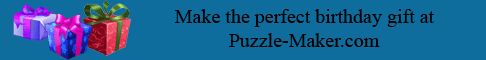Cliches 3--When you're not having any success

```
1. C F M B   K X   P Y I E X   V S D   W S B ' X   E D A A M M W ,

X I V   X I V   K L K Y B
```

```

```
```2. C F M B   X F M   L S Y B L   L M X E   X S D L F ,   X F M

X S D L F   L M X E   L S Y B L
```

```
```
```3. I S T M   C K E   B S X   Q D Y N X   Y B   S B M   W K V
```

```
4. Y P   K X   P Y I E X   V S D   W S B ' X   E D A A M M W ,

W M E X I S V   K N N   M H Y W M B A M   X F K X

E D L L M E X E   V S D   X I Y M W
```

```
5. X F M   I S K W   X S   E D A A M E E   Y E   K N C K V E

D B W M I   A S B E X I D A X Y S B
```

```
6. Y P   K N N   M N E M   P K Y N E ,   T K B Y O D N K X M

X F M   W K X K
```

```
7. Y P   K X   P Y I E X   V S D   W S B ' X   E D A A M M W ,

I M W M P Y B M   E D A A M E E
```